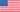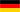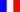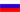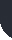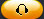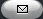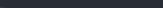Task Management Software for Team Collaboration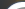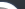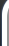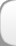HomeDownloadSupportPurchase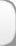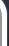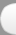Installation GuideStartUp GuideOnline Help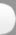# Project Cost Growth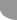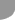Home  » Products  » VIP Task Manager Pro  » Tutorials  » Customization Guide  » Project Cost Growth

Project Cost Growth

Project Cost Growth is a key performance indicator that shows how the actual project cost is changed over time against initial estimated cost. The value is expressed as percentage. Here is the formula to measure project Cost Growth:

Project Cost Growth = (Actual Cost - Baseline Cost) / Baseline Cost

Baseline Cost is initially estimated time-phased budget of a project or some of its phases.

Actual Cost is the total amount that is actually spent in course of the entire project or some of its phases.

Assume that at this stage Baseline Cost of a project is \$5 320 USD. Actual Cost is \$5 900 USD. Current Project Cost Growth is calculated by this equation:

\$5 900 USD - \$5 320 USD / \$5 320 USD = 10.90 %

The negative value speaks about good performance. The project cost is under budget.

If the value for Project Cost Growth is positive, the project is over budget.

Baseline Cost and Actual Cost components vary with business area you are in and your functions. For example, applying to construction project Actual Cost for owners is total actual amount authorized before the project is launched. Actual Cost for contractors is total actual amount spent by the time the project is closed.

Project Cost Growth is one more metric to define whether your project is
over budget , on budget or under budget.
To calculate Project Cost Growth follow the instructions below:

• Add the custom field Baseline Cost with Code “cf_baseline_cost” and Type “Currency”. You may use the following formula to calculate Baseline Cost:

Baseline Cost = (Baseline Work * Standard Rate) + (Baseline Overtime Work * Overtime Rate) + Resource per Use Cost + Task Fixed Cost

All values in this equation are estimated amounts.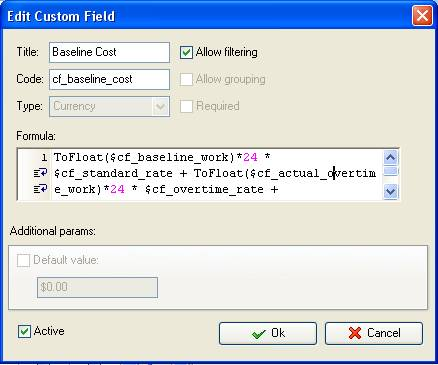• Add the custom field Actual Cost with code “cf_actual_cost” and Type “Currency”. Keeping with the formula above you may use similar formula (all values are actual amounts):

Actual Cost = (Actual Work * Standard Rate) + (Actual Overtime Work * Overtime Rate) + Resource per Use Costs + Task Fixed Cost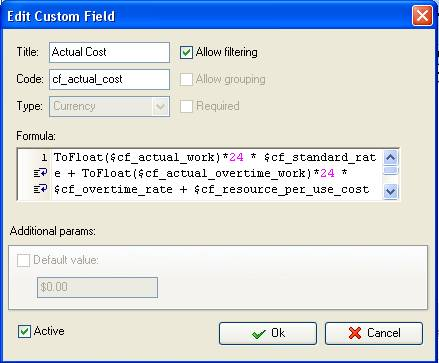• Add the custom field “Cost Growth” with the formula:

(\$cf_actual_cost - \$cf_baseline_cost)/ \$cf_baseline_cost * 100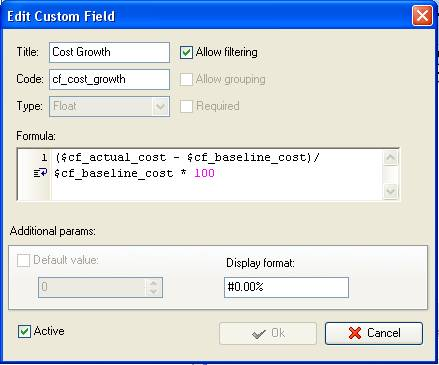• On Task Tree select the project task group for which you need to display project Cost Growth and select “Custom fields options”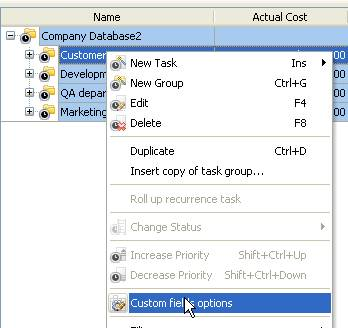• Set the following custom fields settings for the custom field “Cost Growth”

- In “Settings for” select “This task group”
- select “Enable”
- select “Calculated by custom field formula (for task group)”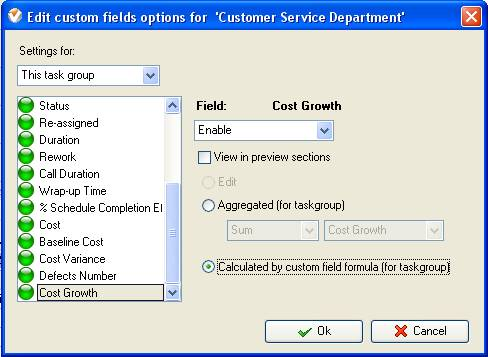If the custom fields “Baseline Cost” and “Actual Cost” are also calculated by the formulas the custom field settings per task group are the same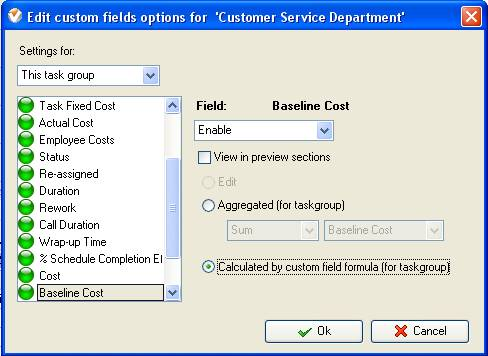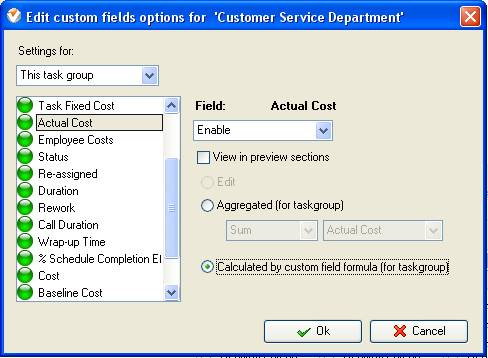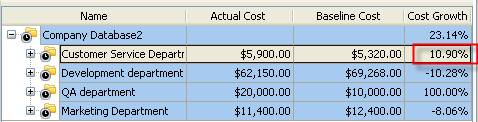Project Cost Growth on Task Tree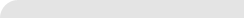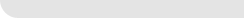» VIP Task Manager Pro VIP Task Manager Std Testimonials Turnkey Solution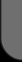ProductsSolutionsDownloadSupportPurchaseContactsForumVideoMy Account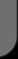Copyright © 2004 - 2023 Task Management Software